#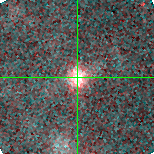M33-013242.26 (J013242.26+302114.1)

## Target Notes### Classification: B[e]sg

Not resolved from J013242.31+302113.9 of comparable brightness less than one arcsecond SE.

## Plots of Brightness and Color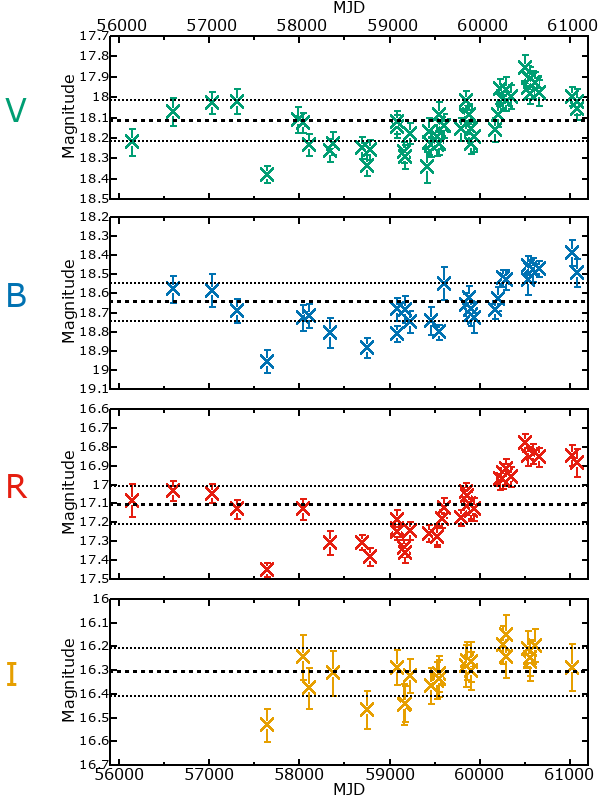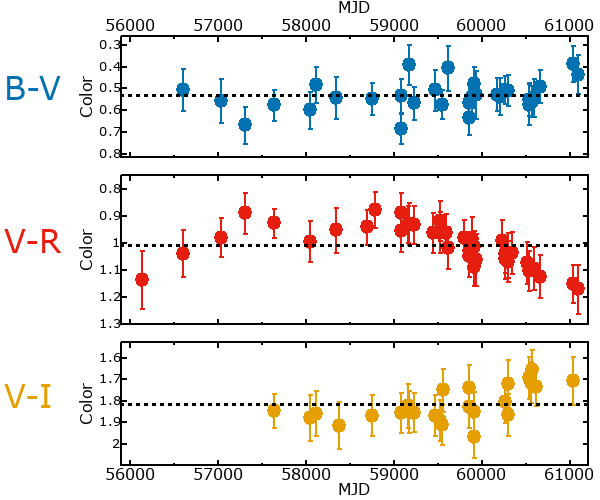## Variability

It is very likely that this star is variable.

### Correlation factors between bands

If the brightness variation is true variability then there should be a correlation between brightness changes recorded in different filter bands. The following table are the R-squared linear correlation factors calculated for the pairs of bands. The number in parentheses next to the R-squared value is the number of elements used to calculate the correlation factor. The number in square brackets next to the band is the range of magnitudes measured.

V [0.40 mag]B [0.37 mag]R [0.46 mag]
B [0.40 mag]0.60 (12)
R [0.46 mag]0.77 (14)0.69 (10)
I [0.28 mag]0.93 (9)0.54 (7)0.98 (6)

### Magnitude-Magnitude Plots

The plots below are provided to check the values in the table above and also to show any non-linear correlations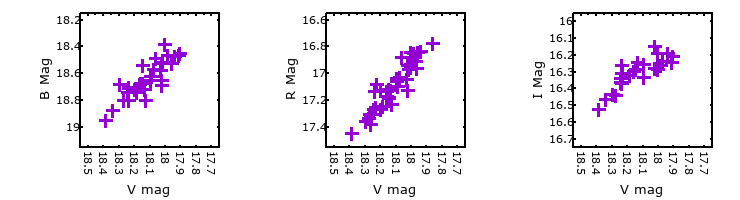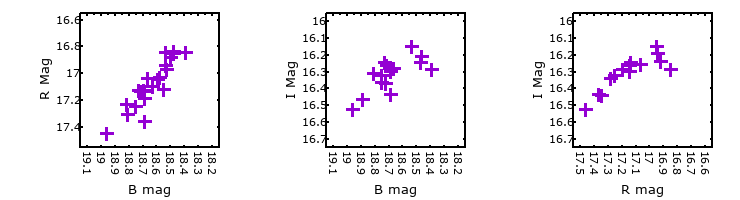### I & R Statistics

Welch & Stetson (1993, AJ, 105, 1813) present a method for varible star detection in CCD images from automated surveys. Data from two bands are compared and two statistics are calculated: R and I.

The I-statistic has an expectation value of zero for stars that are NOT variable. A larger non-zero I-value indicates a higher likelihood that the star is variable. The R statistic indicates how the color of the star changes as the variable grows brighter with R = 1.0 being no change in color.

R and I values have only been calculated if there are more than four (4) matched measurements of brightness in each band.
V
(I|R)
B
(I|R)
R
(I|R)
B
(I|R)
3.31 | 0.73
R
(I|R)
5.74 | 0.745.41 | 0.04
I
(I|R)
2.03 | 1.401.73 | 1.363.35 | 1.76